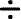Skill 13E
Algebra: Use Related Multiplication and Division
A related division sentence for A x 4 = 12 would be 12 divided by 4 = A. They are related because they both use the same numbers and one helps solve the other. The answer would then be A = 3 since 12 divided by 4 = 3. The related division sentence is 12 divided by 4 = 3.
You probably noticed that this is the basically the same as fact families.
 __________ = _____